# Circle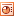3D Circles Grab some real life cones, cylinders, spheres, prisms (sellotape, a tin can, football, tennis ball etc) and print these templates with formulae prompts, for students find the volumes.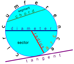Circles Words One page powerpoint of words to know all about circles. Print version.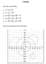Circles Equations Worksheet to check understanding of association of graph of circle to its equation. PDF here.Pi Set of slides that I use when introducing circles. As a slideshow or as a Word doc. Includes Pi as shown here, to 8dp with a catchy phrase, to 30dp and to 100's of dp just for fun! (see below). More phrases created by pupils to remember Pi.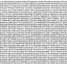Pi to 50,000 dp Yes, its Pi to 50,000 decimal places! Just because I found it (on a link from the Dr Math website) and just because we can! Its a Word doc, 14 or 15 pages long, size 12pt font. Should get across the idea that Pi does indeed go on forever!Circle Links Flowchart linking circle formulae for radius, diameter, circumference, and area. Forwards and backwards. Print version.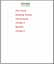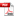Circles Lots of activities involving circle formulaArea of Circles Demonstrates nicely by making you consider why the formula for the area of a circle is what it isISS Earth Circumference KS3 and GCSE, circles (circumference formula) activity. Real life application of circumference of a circle formula to work out the circumference of the Earth using altitude, speed and orbital time of the International Space Station.  A good extension task is to then find percentage error between this answer and actual answer.Circle Questions Word questions about circle area & circumference using formulae forwards & 'backwards'. Leads niceley into ISS Earth Circumference.More Circle Questions More questions about circle area & circumference, pictoral based this time.Mixed Circle Questions A mix of questions about circle area & circumference using formulae forwards & 'backwards'.The Fairy Godmothers Magical Circles Practice Not really written by the fairy godmother and not very magical either, but it sure is circles practice!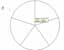Circle Sectors Print off a circle, or set of circles, with as many sectors as you choose (1 - 20+) by using the spinner. Useful for circle topics, pie charts and others.Circle Theorems A talk through show of the 5 'angles in circles' facts that pupils need to know.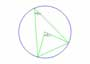Cabri File: Circle Theorems - Angles at Centre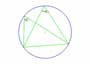Cabri File: Circle Theorems - Angles on CircumferenceCabri File: Circle Theorems - Angles on Diameter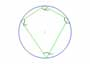Cabri File: Circle Theorems - Cyclic QuadrilateralsCabri File: Circle Theorems - Tangents